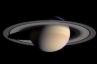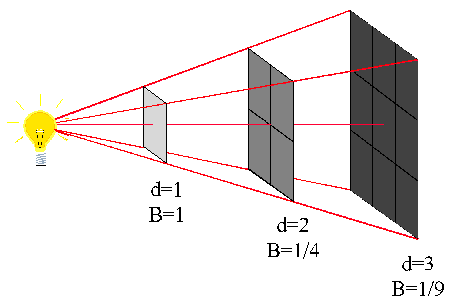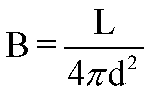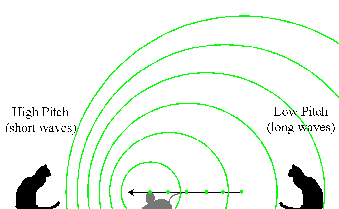Astronomy 161: An Introduction to Solar System Astronomy Prof. Richard Pogge, MTWThF 9:30

# Lecture 22: Light the Messenger

## Key Ideas:

Light is Electromagnetic Radiation
Light as Waves or Photons
Light carries energy at the speed of light
• Waves, or
• Particles (Photons)

The Electromagnetic Spectrum
Sequence of photon energies

Luminosity vs. Apparent Brightness
Inverse Square Law of Brightness

Doppler Effect
Due to relative motion between source & observer
Way to measure speeds at a distance

• ## Electromagnetic Radiation

Light is Electromagnetic Radiation, a self-propagating Electromagnetic disturbance that carries energy at the speed of light

Two complementary ways to describe light

• Electromagnetic Waves
• Photons (particles of light)

## Wave Nature of Light

Can describe light as Electromagnetic Waves

• Periodic (repeating) changes in the strengths of coupled electric and magnetic fields.
• Electromagnetic waves travel through a vacuum at the speed of light.
• Doesn't need a medium to "wave" in.
The speed of light (c) is the same for all light waves:

c = 299,792.458 km/sec

This speed is independent of the wavelength or frequency of the light wave!

## Particle Nature of Light

We can also describe light as a stream of particles or Photons.

Photon:

Massless particles that carry energy at the speed of light.

Photons are characterized by their Energy, which is proportional to their Frequency, f.

Photon Energy:

E = hf
where: f = frequency of the light, and h = Planck's Constant

In words:

## Higher Frequencies mean Higher Energies

Note: Energy, E, is a "particle property", whereas frequency, f, is a "wave property". They are related through Planck's Constant, h. Planck's constant is one of the "Fundamental Constants" of Nature (another one we've met in this lecture is the speed of light, c). One can think of Planck's constant as serving as the "link" between the wave and particle natures of light, and indeed h is the fundamental constant in "quantum mechanics", the modern view of matter and radiation as entities ("quanta") that have the properties of both waves and particles.

## The Electromagnetic Spectrum

The sequence of photon energies running from low energy to high energy is called the Electromagnetic Spectrum

low energy = low frequency = long wavelength

Examples:

high energy = high frequency = short wavelength

Examples:
Ultraviolet, X-rays, Gamma Rays

## Major Divisions of the Electromagnetic Spectrum

 Type of Radiation Wavelength Range Gamma Rays <0.01 nm X-Rays 0.01-10 nm Ultraviolet 10-400 nm Visible Light 400-700 nm Infrared 700-105 nm (0.1 mm) Microwaves 0.1-10mm Radio >1 cm

## The Visible Spectrum

This is all forms of light we can see with our eyes.
Wavelengths: 400 - 700 nanometers (nm)
Frequencies: 7.5x1014 - 4.3x1014 waves/second

We sense visible light of different energies as different colors. The basic colors of the visible spectrum are defined roughly as follows, in order of increasing photon energy:

 Color Name Red Orange Yellow Green Blue Indigo Violet Approximate Wavelength 700nm 650nm 600nm 550nm 500nm 450nm 400nm

You can remember the order of these colors from lowest to highest energy using the famous mnemonic: ROY G. BIV

Important Note:

The wavelengths given in the table above are only approximate. Color is a physiological response of our brains to visible light of different energies. Our division of the visible spectrum into named colors is very subjective, and certainly not a matter of even divisions every 50 nm of wavelength! I include approximate wavelengths primarily for purposes of illustration. An oft-cited example of the subjectivity of color names is the color "orange". It did not enter the language until the Middle Ages, when the fruit of the same name reached Europe from the Middle East. Before that, the color would have been called a reddish shade of yellow.

## How "Bright" is a Light Source?

We need to quantify how bright a light source is. The most convenient way is using the photon picture for light:
• "Brightness" measures the number of photons per second emitted by the light source
There are two ways to quantify this:
Luminosity (L):
Measure of the total energy output:
• L is measured in Power Units (energy/sec) like Watts
• L is independent of distance
Luminosity is an intrinsic property of the light source.

Apparent Brightness (B):
Measures how bright an object appears to be as seen from a distance
• B is measured in Flux Units (energy/sec/area)
• B depends on the distance to the source
Brightness is what we actually measure (an observable property).

## The Inverse Square Law of Brightness

As light travels away from its source, the photons get spread out over increasingly greater areas. The more the light is spread out, the fainter it appears in any given patch of area (for example, the small patch of area covered by the opening in your eye):This means that the Apparent Brightness, B of a light source with intrinsic Luminosity L will get fainter as the square of our distance from the source:In words:
Apparent Brightness is inversely proportional to the square of the distance to the source
Implications:
If the source is 2x closer, it appears 4x brighter.
If the source is 2x farther away, it appears 4x fainter.
This law is extremely important to us in astronomy.

## The Doppler Effect

The observed wavelength of a wave will change when the source of the waves and the observer are moving either towards or away from each other.

Examples:

• Sound Waves (Siren or Train Horn)
• Light Waves
The amount of the shift and its sign depends on
• relative speed of the source & observer
• direction of motion (together or apart)

## Doppler Effect in Sound

Two cats are sitting between a windup mouse toy that emits an electronic squeak. The mouse is moving towards the left towards the first cat and away from the second:The mouse emitted a squeak when it was at the location of each of the green dots. The sound wave ("squeak!") moves outward spherically from each point of emission. Because the mouse is moving, the sound waves have different emission centers. Those waves ahead of its motion (towards the left) are are scrunched together, while those behind are spread out.

Results:

• The cat on the left hears a higher-pitched squeak because the waves have a shorter wavelength (scrunched together by the mouse's motion).

• The cat on the right hears a lower-pitched squeak because the waves have a longer wavelength (spread out by the mouse's motion).

## Doppler Effect in Light

The Doppler Effect in light works the same way as it does for sound:
• Light source moving away from the observer, the observed wavelength will get longer, and hence REDSHIFTED

• Light source moving towards the observer, the observed wavelength will get shorter, and hence BLUESHIFTED

## A Way to Measure Speeds

Observe the wavelength lobs of a light source with a known emitted wavelength lem.

The difference between the observed and emitted wavelengths is directly proportional to the speed of the source towards or away from you (v), given by the Doppler Formula:Here c is the speed of light.
• The size of the shift gives the speed of the source
• The color of the shift (Red or Blue) gives the direction of motion (away or towards you).

## The Doppler Effect in Practice

The Doppler Effect in light is used by astronomers to measure the speeds of objects moving towards or away from the Earth.

But, we also use the Doppler Effect in light in everyday settings. Some examples:

Radar gun bounces a pulse of microwaves (or infrared laser light) of a known wavelength off a car or truck, measure the wavelength reflected back. The Doppler shift gives the vehicle's speed.

Similar principle, bounce microwave radar signals of known wavelength off of clouds, measure the wavelength reflected back. The Doppler shift and its sign (blue or red) gives the speed and direction of the clouds. The strength of the returned signal also gives the amount of rain or snow falling.

## Light the Messenger

The properties of light give us a way to bridge the vast distances between us and astronomical objects.

The light "encodes" important information about the source of the light

1. Luminosity measures how much energy the object emits in the form of light.
2. Doppler Shift tells us how fast it is moving towards or away from us
To learn more from light, however, we need to understand how light and matter interact, which is the subject of next few lectures.
Return to [ Unit 4 Index | Astronomy 161 Main Page ]
Updated: 2006 October 21